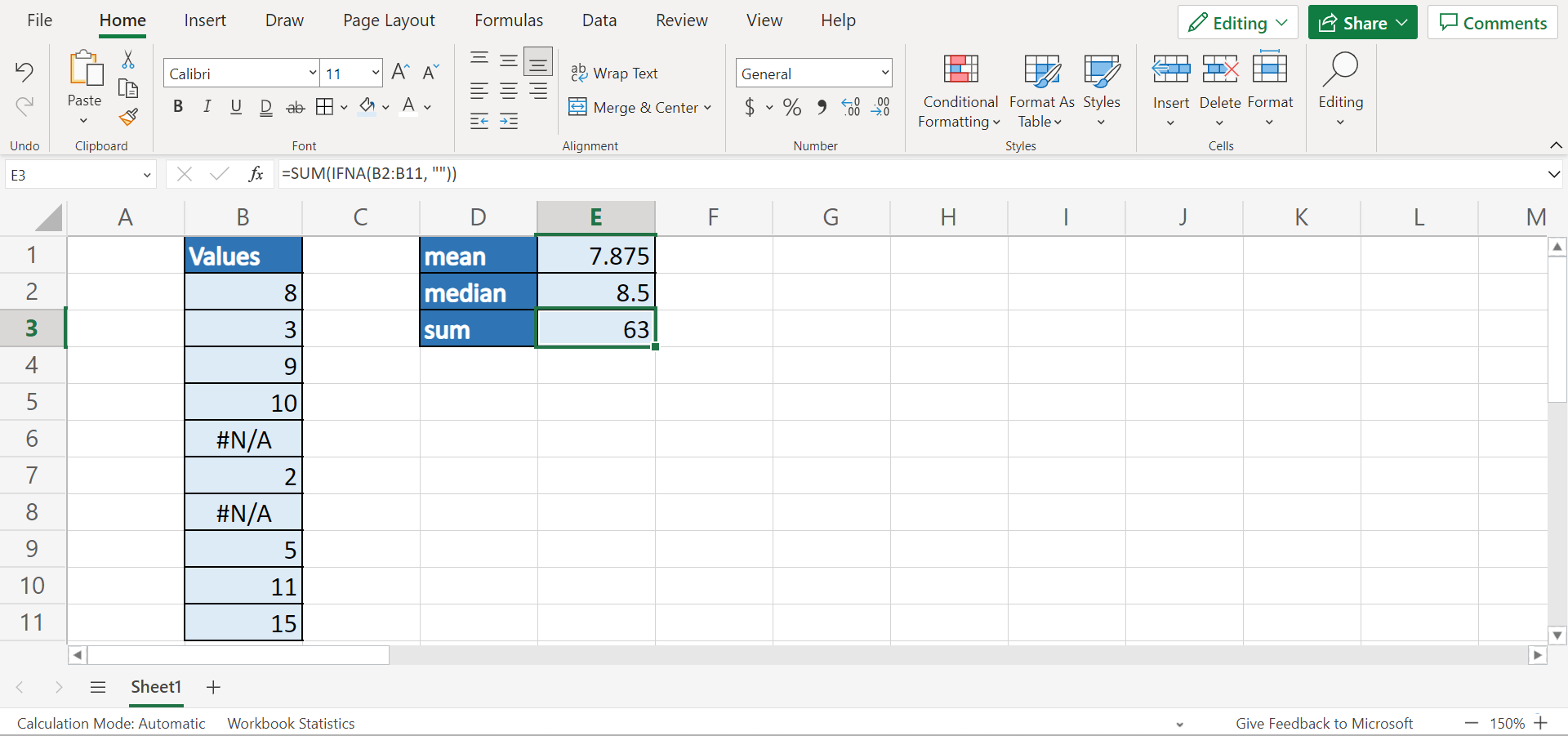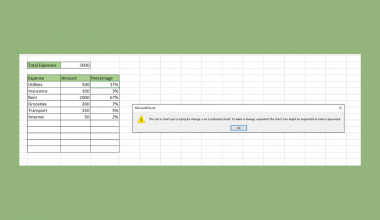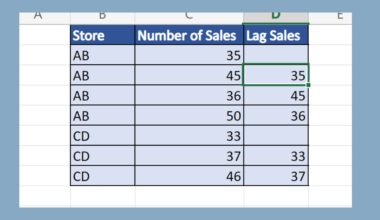# How to Ignore #N/A Values in Excel

This guide will discuss how to ignore #N/A values in Excel using the `IFNA` function

The rules for using the `IFNA` function in Excel are the following:

• The `IFNA` function is an easy way to trap and handle #N/A errors without catching other errors.
• When the value argument is empty, the function will evaluate it as an empty string, not an error.
• When the value_if_na argument is inputted as an empty string, the function will return blank, or display no message when an error is detected.
• The function only traps #N/A errors and provides an alternative result.

Excel is an excellent tool to use for different purposes and situations. Since it has several built-in functions and tools, we can easily perform complex and long calculations. However, we cannot avoid mistakes or errors as we do calculations in Excel.

The great thing about Excel is that it would return warnings such as #N/A errors to remind us if we ever made a mistake in our calculations. And this helps out whenever we perform long calculations because we can immediately point out where the calculation went wrong.

But, sometimes we just want to ignore the errors and continue with our calculations. In this guide, we will focus on learning how to ignore #N/A values in Excel.

So a #N/A value is an error value that means no value is available for that particular cell. Some people use the #N/A value to mark empty cells to avoid accidentally including empty cells in the calculations.

Luckily, there are several methods we can use to ignore #N/A values in Excel and continue with our calculations. For instance, we can utilize the `IFNA` function to ignore the #N/A values and proceed with our needed calculation.

Let’s take a sample scenario wherein we need to ignore #N/A values in Excel.

Suppose you have a data set containing the #N/A error values. However, you still want to proceed and calculate the data set’s mean. To do this, you used the `AVERAGE` function together with the `IFNA` function.

With this formula, you could replace the #N/A values with blanks and then calculate the mean.

Before we move on to a real example of ignoring #N/A values in Excel, let’s first learn how to use the `IFNA` function.

## The Anatomy of the IFNA Function

The syntax or the way we write the `IFNA` function is as follows:

`=IFNA(value, value_if_na)`

Let’s take apart this formula and understand what each term means:

• = the equal sign is how we begin any function in Excel.
• IFNA() is our `IFNA` function. And this function is used to return the value we specify if the expression resolves to #N/A. Otherwise, the function will return the result of the expression.
• value is a required argument. So this refers to any value or expression, or cell reference.
• value_if_na is another required argument. And this refers to any value or expression, or cell reference.

Great! Now we can move on and dive into a real example of ignoring #N/A values in Excel.

## A Real Example of Ignoring #N/A Values in Excel

Let’s say we have a data set containing multiple #N/A error values. However, we still want to proceed to perform different statistical calculations. So our initial data set would look like this:For instance, we need to calculate the mean, median, and sum of the data set. However, the data set contains several #N/A error values. Luckily, we can still perform the calculation while ignoring the #N/A error values using the `IFNA` function.

So the `IFNA` function traps and handles #N/A errors without catching other errors such as #NAME? errors. Furthermore, the function will return a custom result when the formula generates a #N/A error. Otherwise, it will return a normal result when no error is detected.

But, the `IFNA` function will only handle #N/A errors. So other errors will still be displayed when it is found.

In this case, we will combine the functions we will use for calculations with the `IFNA` function. For example, we want to calculate the mean of our data set. And we will simply combine the `AVERAGE` function with the `IFNA` function.

So we will let the `IFNA` function simply replace the #N/A values with blanks. Then, we will continue to calculate the mean using the `AVERAGE` function. Furthermore, we will use the same method when doing other calculations.

For instance, we also want to get the median of our data set. So we can simply combine the `MEDIAN` function with the `IFNA` function. Similarly, the formula will convert the #N/A errors to blanks and continue with the calculation. In this case, it will return the median.

Lastly, we also want to calculate the sum of the data set while ignoring the #N/A error values. To do this, we will combine the `SUM` and `IFNA` functions.

Once again, the `IFNA` function will change the #N/A error values to blanks. Then, the `SUM` function will proceed to calculate the sum of the values in the data set. So our final data set would look like this:Amazing! Now we can proceed and discuss how to ignore #N/A values in Excel.

## How to Ignore #N/A Values in Excel

In this section, we will discuss the step-by-step process of how to ignore #N/A values in Excel using the `IFNA` function. Furthermore, each step contains detailed instructions and pictures to guide you along the way.

To apply this method to your work, we can simply follow the steps below.

1. Firstly, let’s calculate the data set’s mean while ignoring the #N/A error values. To do this, we can simply type in the formula “=AVERAGE(IFNA(B2:B11, “”))”. Then, we will press the Enter key to return the result.2. Secondly, we will calculate the median while ignoring the #N/A error values. To do this, we will input the formula “=MEDIAN(IFNA(B2:B11, “”))”. Next, we will press the Enter key to return the result.3. Thirdly, we can try to calculate the sum of the data set while ignoring the #N/A error values. To do this, we can simply type in the formula “=SUM(IFNA(B2:B11, “”))”. Lastly, we will press the Enter key to return the result.4. And tada! We have successfully ignored #N/A error values in Excel.And that’s pretty much it! We have successfully explained how to ignore #N/A error values in Excel using the IFNA function. Now you can use this method and apply it to your work whenever you encounter #N/A error values.

Are you interested in learning more about what Excel can do? You can now use the `IFNA` function and the various other Microsoft Excel formulas available to create great worksheets that work for you. Make sure to subscribe to our newsletter to be the first to know about the latest guides and tutorials from us.

e=”font-weight: 400;”>Our goal this year is to create lots of rich, bite-sized tutorials for Google Sheets users like you. If you liked this one, you'll love what we are working on! Readers receive ✨ early access ✨ to new content.

##### You May Also Like## How to Create a Covariance Matrix in Excel

This guide will explain how to create a covariance matrix in Excel. The covariance matrix is a useful…## How to Protect Formulas Without Protecting Worksheet in Excel

This guide will explain how you can protect formulas without protecting worksheets in Excel. You can protect cells…## How to Use Less Than or Equal to Operator in Excel

The less than or equal to operator in Excel is useful when you need to know if a…## How to Lookup a Value in a 3D Table in Excel

This guide will explain how you can use Excel functions to lookup values in a 3D table in…